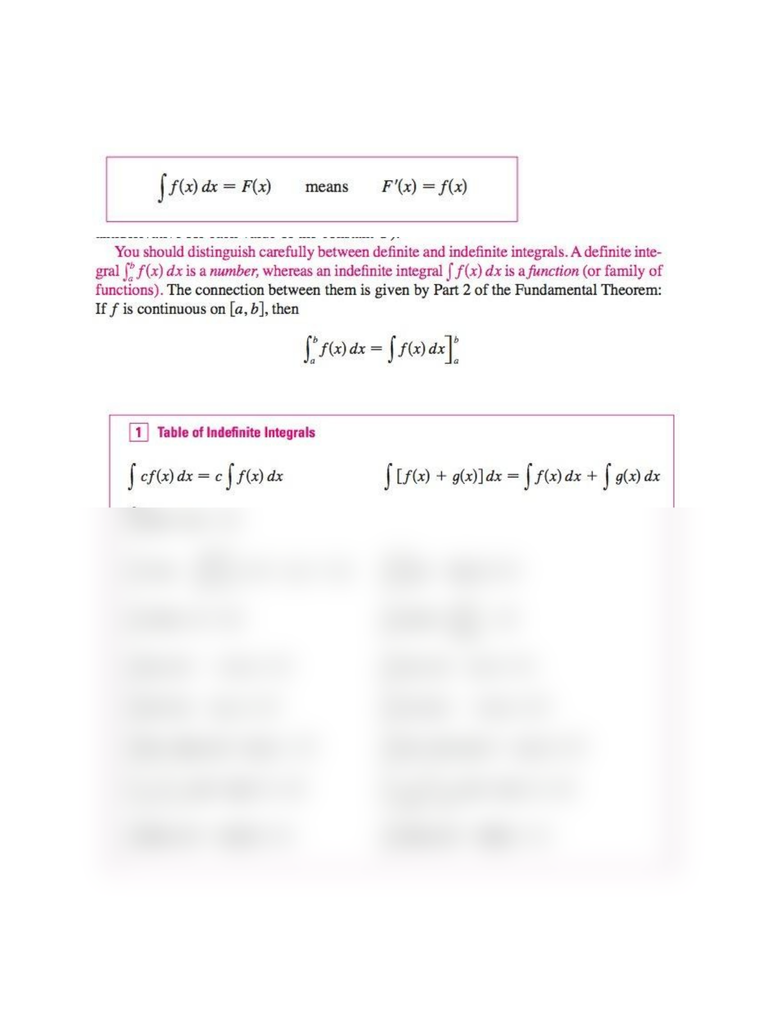Textbook Notes (280,000)
US (110,000)
UCSB (700)
MATH 3B (10)
helms (10)
Chapter 5.4

# MATH 3B Chapter Notes - Chapter 5.4: Antiderivative

This preview shows half of the first page. to view the full 2 pages of the document.Chapter 5.4 Indefinite Integrals and the Net Change Theorem
Indefinite integral: notation ſ f(x) dx used as an antiderivative of f
Antiderivatives of functions